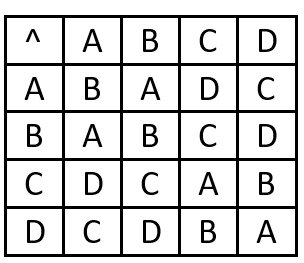# Math Worksheets Land

Math Worksheets For All Ages

# Math Worksheets Land

Math Worksheets For All Ages

Home > Math Topics > Algebra >

# Binary Operation Tables Worksheets

A binary operation is a predetermined rule for how two things combine for a given type and produce a new thing. This rule sets commonly use letters and numbers but can really use any form. We use binary operation tables to chart all the possible outcomes of a binary operation. We will often need to look for imaging trends in these tables and interpret them for others. We are often looking for an identify element existing. You can easily spot those tables because all of the value in a row or column are the same. We will look at other properties that may exist in these tables over the course of these lessons. These worksheets help students learn how to interpret and even create their own binary operation tables.

### Aligned Standard: REI.C.5

• Answer Keys - These are for all the unlocked materials above.

### Homework Sheets

All these sheets focus on the three major conceptual questions that you will need to answer.

• Homework 1 - The colors will help you here, but are not fully necessary.
• Homework 2 - For the example we use numbers to make it more concrete for students.
• Homework 3 - Many teachers have a preference to use letters of the alphabet. We used them for all the tables on this worksheet.

### Practice Worksheets

Over the course of these sheets we are looking for you to better grasp the concepts that are presented.

• Practice 1 - It is a zoo out there! All of these tables are filled with animals.
• Practice 2 - We give you a deep explanation for each of the problems.
• Practice 3 - For any students that are having difficulty, I would send them home with this worksheet and answer key. It will walk them through everything.

### Math Skill Quizzes

There are a ton of different tables for you to interpret here. Make sure to focus your attention on each of the sheets, as needed.

• Quiz 1 - The questions that asks if a statement is true or false give students the most trouble. This is normally because they rush through those problems.
• Quiz 2 - This is mostly numbers and letters for you to work with.
• Quiz 3 - This is a well designed quiz for you to help you determine where your students are at with this skill.

### How to Read Binary Operation TablesThe word binary operation is derived by the word 'bi' which means two i.e. something that is composed of two pieces. A binary operation is a simple rule that combines two values to create a new one. One of the most widely known operations in binary are the ones that we learn in elementary level such as: Addition, subtraction, division and multiplication which are applied on various different numbers.

Binary operation tables are visual depictions of set were all the elements of a binary operation is plotted out. You can see that the table in the above case shows relationship that exists between two identities. The table is an example of a binary operation table where (^) is the binary operation performed on the set {A, B, C, D}. How you go about reading this is to match up the columns, much like a times table. For example, if we were looking for the end value of B^C, we would look at the vertical column for (B) and match it up with the horizontal column (C). We would clearly see that B^C = C.

As we can see these tables give us predetermined outcomes that we can use to interpret the nature of the binary relationship. There are six common properties of these relationships that we have seen before when working with basic operations.

Unlock all the answers, worksheets, homework, tests and more!
Save Tons of Time! Make My Life Easier Now

## Thanks and Don't Forget To Tell Your Friends!

I would appreciate everyone letting me know if you find any errors. I'm getting a little older these days and my eyes are going. Please contact me, to let me know. I'll fix it ASAP.